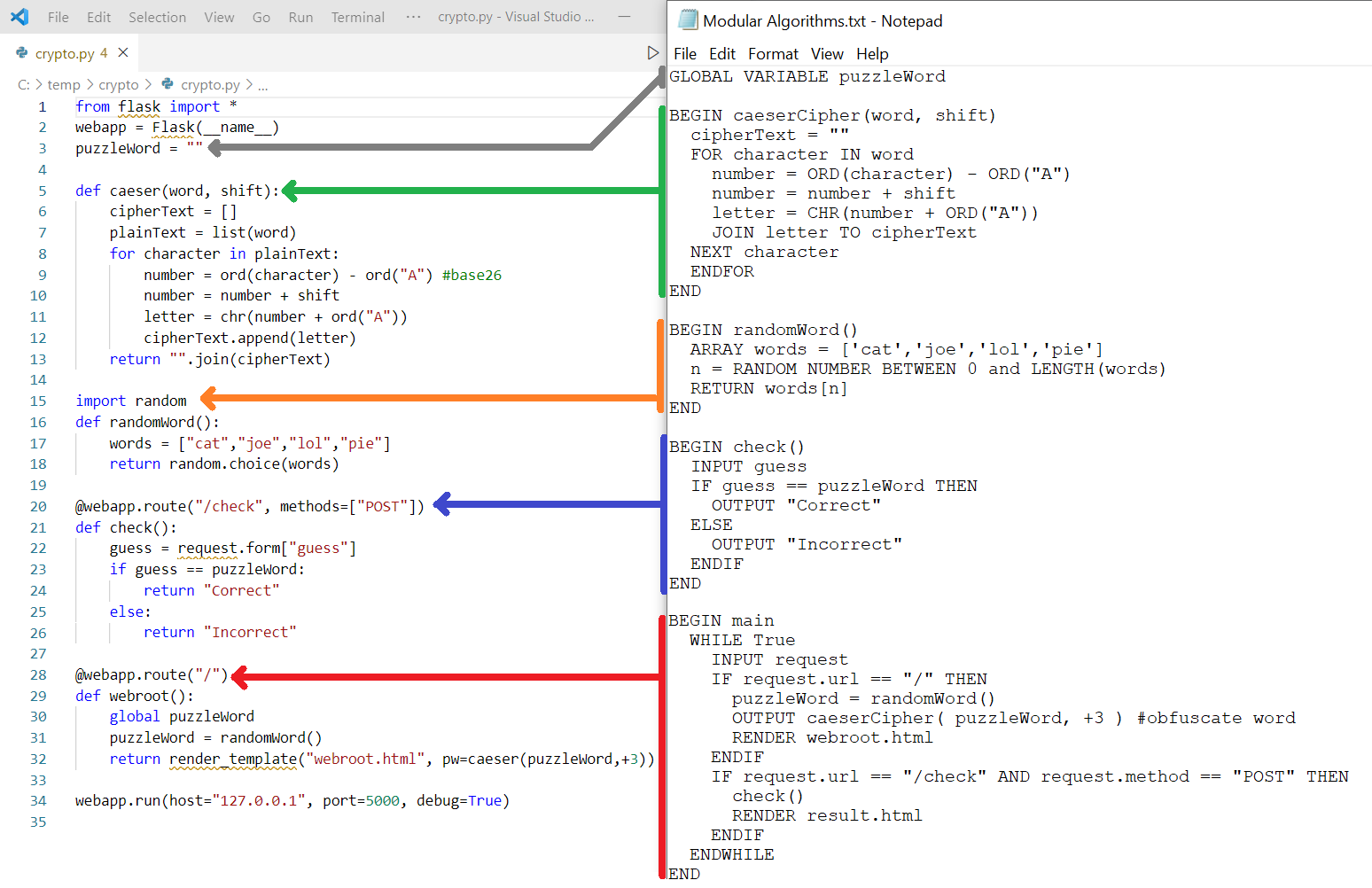#### Algorithms

Steps required to solve a problem.Algorithmic theory for Digital Solutions conventions sequence and assignment selection iteration modularity QCAA materials on algorithms
 Algorithms for Web Servers Basic Algorithm for a Web Server Web Server Algorithm - more complex Algorithms to Coded Components
Practise Exercises - Plan Algorithms & Code
Exercise 1 - Mailing Label | solution
Exercise 2 - Lowest Number | solution
Exercise 3 - Five Stars | solution
Exercise 4 - Award Grades | solution | alt solution
Exercise 5 - Distance To | solution
Exercise 6 - Watch List | solution
 older algorithm theory pseudocode supplemetary materials for algorithms sorts visualised bubble sort insertion sort recursion shuffle merge sort quick sort flow charts big O

```            BEGIN gcd(a, b)
WHILE NOT(b == 0)
temp = a
a = b
b = temp MOD a
ENDWHILE
RETURN a
END

BEGIN
WHILE 1==1